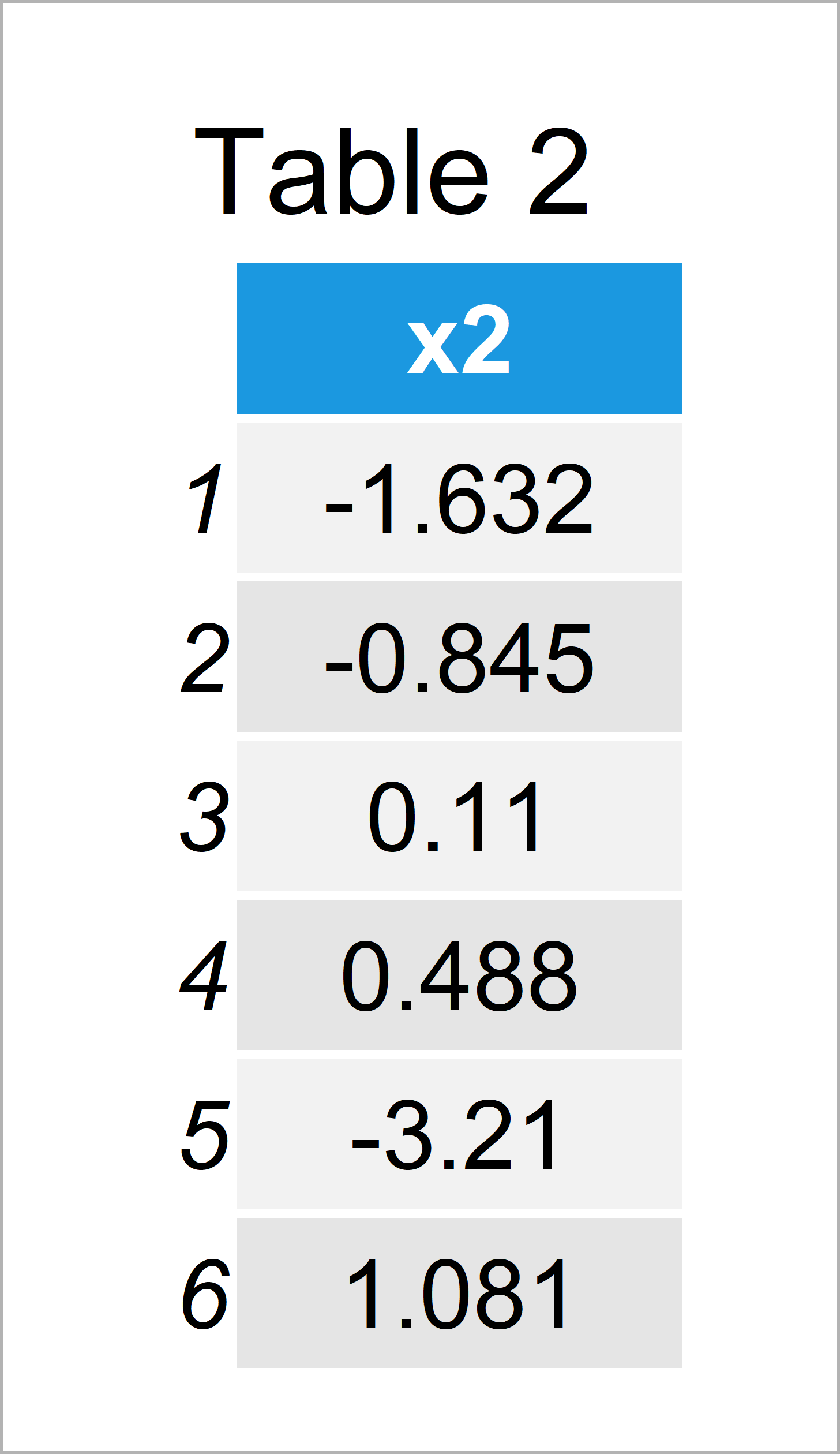# R predict() Warning message: ‘newdata’ had X rows but variables found have Y rows

In this article, I’ll show how to handle the predict function “Warning message: ‘newdata’ had X rows but variables found have Y rows” in R programming.

The content of the tutorial looks like this:

Let’s dive right into the exemplifying R code:

## Creation of Exemplifying Data

The first step is to create some example data. Since we will create random data, we have to set a random seed:

`set.seed(563988727)                    # Set random seed`

Next, we can create our first data frame as shown below:

```x1 <- rnorm(100)                       # Create first data frame
y1 <- rnorm(100) + x1
data1 <- data.frame(x1, y1)
head(data1)                            # Print first data frame```Have a look at the table that got returned after executing the previous R code. It shows that our first example data frame has 100 rows and two columns called x1 and y1.

Let’s create a second data frame:

```data2 <- data.frame(x2 = rnorm(50))    # Create second data frame
head(data2)                            # Print second data frame```After executing the previous syntax the data frame illustrated in Table 2 has been created. This data frame contains only one variable called x2.

Now, we can estimate a linear regression model using our first data frame and the lm function:

`mod <- lm(y1 ~ x1, data1)              # Estimate linear model`

The previous R code has created a model object called mod.

## Example 1: Reproduce the Warning message: ‘newdata’ had X rows but variables found have Y rows

Example 1 shows how to replicate the “Warning message: ‘newdata’ had X rows but variables found have Y rows” in R.

Let’s assume that we want to use our linear model object mod to predict values for the second data frame. For this, we might try to apply the predict function as shown below:

```pred_values <- predict(mod, data2)     # Apply predict function
# Warning message:
# 'newdata' had 50 rows but variables found have 100 rows```

Unfortunately, the RStudio console returns the “Warning message: ‘newdata’ had X rows but variables found have Y rows” after running the previous syntax.

The reason for this is that the column name of our predictor in the second data frame is different from the predictor name in the first data frame (i.e. x2 in the second data and x1 in the first data).

So how can we solve this problem?

## Example 2: Fix the Warning message: ‘newdata’ had X rows but variables found have Y rows

Example 2 illustrates how to deal with the “Warning message: ‘newdata’ had X rows but variables found have Y rows”.

As a first step, we have to harmonize the variable names of data1 and data2. To achieve this, we can simply rename the column name of the predictor in the second data frame:

`colnames(data2) <- "x1"                # Rename column`

Next, we can apply the predict function as we already did before:

```pred_values <- predict(mod, data2)     # Apply predict function

This time it works without any warning messages. The previous output of the RStudio console shows the head of our properly predicted values.

## Video, Further Resources & Summary

I have recently published a video on my YouTube channel, which illustrates the examples of this tutorial. You can find the video below:

Furthermore, you may want to have a look at the other articles of this website. You can find some related tutorials below:

Summary: In this article, I have illustrated how to avoid the “Warning message: ‘newdata’ had X rows but variables found have Y rows” in R. Let me know in the comments section, if you have any further questions.

Subscribe to the Statistics Globe Newsletter